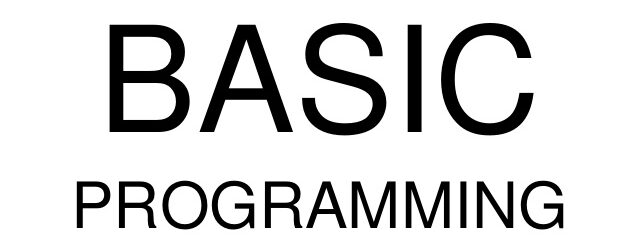# Introduction to BASIC Programming- Use of Parenthesis

Welcome to Class !!

In today’s Computer Science class, We will continue learning about programming in BASIC. We hope you enjoy the class!#### Parentheses Rule

There are cases where the precedence rule may cause a problem. For example:

The BASIC expression LET Y = A/B+ C would produce undesired results because A would be divided by B and the result added to C. The solution to this problem is in the use of parentheses. If we let parentheses override the order of precedence (but maintain the order of precedence within the parentheses), the result will be satisfactory.  Now let’s examine the previous example using parentheses.

Example:

##### Using parentheses

With the parentheses, B is added to C and the sum of this operation is divided into A, giving the correct result.

Sometimes more than one set of parentheses may be needed to tell the BASIC language in what order to execute the arithmetic operations.

Example:

Parentheses inside parentheses

The BASIC expression to accomplish this would be: Let A+B/2^2

For this expression to give us the correct results, A and B must be added first, then the sum divided by C, and finally that result is squared. When parentheses within parentheses are used, the innermost parentheses will be evaluated first. The addition has lower precedence than either division or exponentiation; therefore, A + B must be in the inner parentheses. The division has lower precedence than exponentiation, so (A+B)/C also must be enclosed in parentheses, ((A+B)/C), to ensure it is performed next.

The important thing to remember is the parentheses may be used to override the normal order of precedence.

The parentheses rule says:

• Computations inside parentheses are performed first.
• If there are parentheses inside parentheses, the operations inside the inner pair are performed first.
##### Summary

Simple problems can be solved with BASIC by using only two or three instructions. These are the END, PRINT and LET statements. To use these effectively, you must know how they work and what rules must be followed in using them.

The END statement, which must be the last statement in every BASIC program, has two functions. It indicates to the compiler that there are no more BASIC statements for it to translate and it terminates execution of the program.

The PRINT statement is used to instruct the computer to output something either on the terminal or the printer. The standard print line in BASIC is divided into print zones or fields of 16 spaces each.

The two punctuation marks used in PRINT statements are the comma and semicolon. A comma used as a separator in a PRINT statement causes standard spacing and a semicolon causes packed spacing.

Any Information enclosed in quotation marks in a PRINT statement will be printed exactly as it appears in the program.

The LET statement can be used to assign a constant value to a variable name, a variable to a variable name, or the results of an expression to a variable name. The equal sign in a LET statement does not indicate algebraic equality, rather it means to be assigned the value of. The value assigned by a LET statement is stored in the computer’s memory; therefore, it can be referenced by its variable name.

Both the PRINT and LET statements may contain expressions with arithmetic operations. These arithmetic operations must be specified by the appropriate operation symbol. Should you forget to include the symbol, the computer will not insert it for you but will give you an error message.

Constants and variables are used to refer to numeric values or character strings. A constant is a whole or decimal number or character string whose value does not change. A variable name is an arbitrary name you select and you and the computer used to refer to a value stored in the computer’s memory. This value may vary during the execution of the program but can contain only one value at a time.

###### General evaluation
1. Define a ‘function’?
2. What does the BASIC acronym represent in Programming?
3. State the parenthesis rule.

We have come to the end of this class. We do hope you enjoyed the class?

Should you have any further question, feel free to ask in the comment section below and trust us to respond as soon as possible.

In our next class, we will be learning about Computer Maintainance. We are very much eager to meet you there.

Your Opinion Matters! Quickly tell us how to improve your Learning Experience HERE